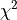# Statistics on sample¶

## Sample¶

 Sample(*args) Sample of real vectors.

## Building distributions from samples¶

 Base class for probability distribution factories. Results of distribution estimation. Result from likelihood estimation. Distribution factory result for profile likelihood estimation. TimeVaryingResult(*args) Distribution factory result for non stationary likelihood estimation. ArcsineFactory(*args) Arcsine factory. BernoulliFactory(*args) Bernoulli factory. BetaFactory(*args) Beta factory. BinomialFactory(*args) Binomial factory. BurrFactory(*args) Burr factory. ChiFactory(*args) Chi factory. ChiSquareFactory(*args) Chi-Square factory. DiracFactory(*args) Dirac factory. DirichletFactory(*args) Dirichlet factory. ExponentialFactory(*args) Exponential factory. Fisher-Snedecor factory. FrechetFactory(*args) Frechet factory. GammaFactory(*args) Gamma factory. GeneralizedExtremeValue factory. Generalized Pareto factory. GeometricFactory(*args) Geometric factory. GumbelFactory(*args) Gumbel factory. HistogramFactory(*args) Histogram factory. Inverse Normal factory. KernelSmoothing(*args) Non parametric continuous distribution estimation by kernel smoothing. LaplaceFactory(*args) Laplace factory. Least squares factory. LogisticFactory(*args) Logistic factory. LogNormalFactory(*args) Lognormal factory distribution. LogUniformFactory(*args) Log Uniform factory. Maximum likelihood factory. Meixner Distribution factory. Estimation by method of moments. MultinomialFactory(*args) Multinomial factory. Negative Binomial factory. NormalFactory(*args) Normal factory. ParetoFactory(*args) Pareto factory. PoissonFactory(*args) Poisson factory. Estimation by matching quantiles. RayleighFactory(*args) Rayleigh factory. RiceFactory(*args) Rice factory. SkellamFactory(*args) Skellam factory. StudentFactory(*args) Student factory. TrapezoidalFactory(*args) Trapezoidal factory. TriangularFactory(*args) Triangular factory. Truncated Normal factory. UniformFactory(*args) Uniform factory. UserDefinedFactory(*args) UserDefined factory. VonMisesFactory(*args) VonMises factory. WeibullMinFactory(*args) WeibullMin factory. WeibullMaxFactory(*args) WeibullMax factory.

## Building copulas from samples¶

 AliMikhailHaq copula factory. BernsteinCopula copula factory. Clayton Copula factory. Farlie Gumbel Morgenstern Copula factory. FrankCopulaFactory(*args) Frank Copula factory. Gumbel Copula factory. Independent Copula factory. Normal Copula factory. Plackett Copula factory.

## Sensitivity Analysis¶

 Correlation analysis methods. ANCOVA(*args) ANalysis of COVAriance method (ANCOVA). FAST(*args) Fourier Amplitude Sensitivity Testing (FAST). Sensitivity analysis. Sensitivity analysis using Martinez method. Sensitivity analysis using Saltelli method. Sensitivity analysis using Jansen method. Sensitivity analysis using MauntzKucherenko method. Experiment to computeSobol' indices. Sobol indices computation using iterative sampling. Sobol simulation result.

## HSIC Indices¶

 HSICStat(*args) Base class of HSICStat. HSICUStat(*args) Unbiased HSIC statistics. HSICVStat(*args) Biased HSIC statistics. HSICEstimator(*args) Base class of HSIC estimators. Implement a HSIC estimator for conditional analysis. Implement a HSIC estimator for global analysis. Implement a HSIC estimator for target analysis.

## Statistical tests¶

 TestResult(*args) Test result data structure.

### Goodness-of-fit metrics & tests¶

 FittingTest.AIC(*args) Compute the Akaike information criterion. FittingTest.AICC(*args) Compute the Akaike information criterion (with correction for small data). FittingTest.BIC(*args) Compute the Bayesian information criterion. Perform agoodness-of-fit test for 1-d discrete distributions. FittingTest.Kolmogorov(sample, distribution) Perform a Kolmogorov goodness-of-fit test for 1-d continuous distributions. FittingTest.Lilliefors(sample, factory[, level]) Perform a Lilliefors goodness-of-fit test for 1-d continuous distributions. Evaluate whether a sample follows a normal distribution. NormalityTest.CramerVonMisesNormal(sample[, ...]) Evaluate whether a sample follows a normal distribution.

### Graphical tests¶

 VisualTest.DrawPairs(sample) Draw 2-d projections of a multivariate sample. VisualTest.DrawPairsMarginals(sample, ...) Draw 2-d projections of a multivariate sample plus marginals. VisualTest.DrawParallelCoordinates(...[, ...]) Draw a parallel coordinates plot. Draw an Henry plot. Draw kendall plot. Plot a 1D linear model. Plot a 1D linear model's residuals. Draw a QQ-plot. Draw a CDF-plot. Draw the upper tail dependence function. Draw the upper extremal dependence function. Draw the lower tail dependence function. Draw the lower extremal dependence function. Validation of GeneralizedExtremeValue inference.

### Hypothesis tests¶

 HypothesisTest.ChiSquared(firstSample, ...) Test whether two discrete samples are independent. HypothesisTest.LikelihoodRatioTest(...[, level]) Nested likelihood model selection. HypothesisTest.FullPearson(firstSample, ...) Test whether two discrete samples are independent. HypothesisTest.FullSpearman(firstSample, ...) Test whether two samples have no rank correlation. HypothesisTest.PartialPearson(firstSample, ...) Test whether two discrete samples are independent. HypothesisTest.PartialSpearman(firstSample, ...) Test whether two sample have no rank correlation. HypothesisTest.Pearson(firstSample, secondSample) Test whether two discrete samples are independent. HypothesisTest.Spearman(firstSample, ...[, ...]) Test whether two samples have no rank correlation. HypothesisTest.TwoSamplesKolmogorov(sample1, ...) Test whether two samples follows the same distribution.

### Linear model tests¶

 Test the nullity of the linear regression model coefficients. Test zero mean value of the residual of the linear regression model. Test the homoskedasticity of the linear regression model residuals. Test the homoskedasticity of the linear regression model residuals. Test the autocorrelation of the linear regression model residuals. LinearModelTest.FullRegression(firstSample, ...) Test whether two discrete samples are not linear. LinearModelTest.PartialRegression(...[, level]) Test whether two discrete samples are independent.

### Model selection¶

 Select the best model according to the Akaike information criterion. Select the best model according to the Akaike information criterion with correction. Select the best model according to the Bayesian information criterion. Select the best model according to thegoodness-of-fit test. FittingTest.BestModelKolmogorov(sample, ...) Select the best model according to the Kolmogorov goodness-of-fit test. FittingTest.BestModelLilliefors(sample, ...) Select the best model according to the Lilliefors goodness-of-fit test.